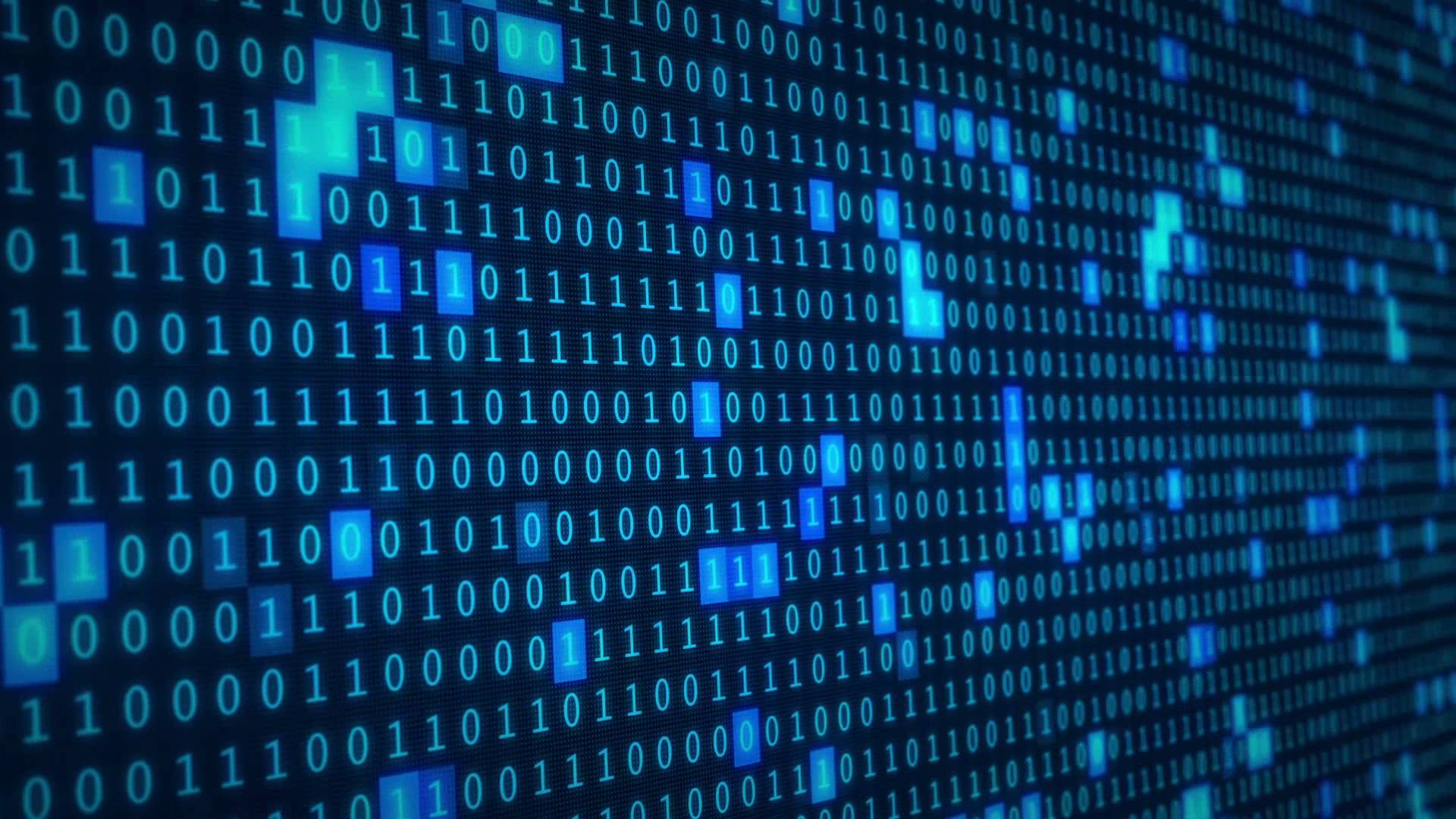Binary code

What is binary code?

Simply defined binary code is a coding system using the binary digits 0 and 1 to represent a letter, digit or other character in an electronic device. This code is used to write data such as the instructions that computer processors use or the digital text you read everyday.

How does binary code work?

As we discussed earlier the zeros and ones are used to represent off and on. Binary numbers use the same rules as decimal - the value of any digit always depends on its position in the whole number.

Decimal uses base ten, so that every time a number moves one position to the left in a figure, it increases by a power of ten (eg. 1, 10, 100 etc). However, binary uses base of two, so each move to the left increases the value by a power of two (eg. 1, 2, 4 etc). This is the basic description of binary code and how it works.